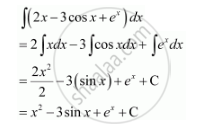Share

# Find the Following Integrals Int(2x- 3cos X +E^X) Dx - CBSE (Commerce) Class 12 - Mathematics

ConceptIntegration as an Inverse Process of Differentiation

#### Question

Find the following integrals int(2x- 3cos x +e^x) dx

#### SolutionIs there an error in this question or solution?

#### Video TutorialsVIEW ALL 

Solution Find the Following Integrals Int(2x- 3cos X +E^X) Dx Concept: Integration as an Inverse Process of Differentiation.
S